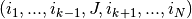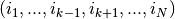# `tensorly.cp_tensor`.cp_mode_dot

`cp_mode_dot`(cp_tensor, matrix_or_vector, mode, keep_dim=False, copy=False)[source]

n-mode product of a CP tensor and a matrix or vector at the specified mode

Parameters: cp_tensortl.CPTensor or (core, factors) matrix_or_vectorndarray1D or 2D array of shape `(J, i_k)` or `(i_k, )` matrix or vectors to which to n-mode multiply the tensor modeint CPTensor = (core, factors)mode-mode product of tensor by matrix_or_vector * of shapeif matrix_or_vector is a matrix * of shapeif matrix_or_vector is a vector

`kruskal_multi_mode_dot`